American Journal of Chemical Engineering
Volume 3, Issue 4, July 2015, Pages: 52-57

Study on Dynamics of Saponification Process Using State Space Model

Khalid Mokhlif Mousa, Zainab Essaim Dawood

Department of Chemical Engineering, Al-Nahrain University, Baghdad, Iraq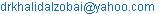(K. M. Mousa)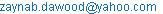(Z. E. Dawood)

Khalid Mokhlif Mousa, Zainab Essaim Dawood. Study on Dynamics of Saponification Process Using State Space Model.American Journal of Chemical Engineering.Vol.3, No. 4, 2015, pp. 52-57. doi: 10.11648/j.ajche.20150304.11

Abstract: Due to multi input multi output variables affected the continuous stirred tank reactor its worthy to use the state space model to study the dynamics of this system. In this work, a saponifecation process was studded on which Ethel acetate was reacted with sodium hydroxide to produced sodium acetate and ethanol. one litter operation volume CSTR was used, the flow rate of Ethel acetate and cooling water were toke as disturbance variables and the concentration of sodium acetate and the temperature of reactor as response variables. The results show improvement compared with linearized model.

Keywords: CSTR, Sodium acetate, State Space Model

Contents

1. Introduction

Continuous stirred tank reactors (CSTR) are the most basic of the continuous reactors used in chemical processes. Continuous stirred-tank reactors (CSTRs) are open systems, where material is free to enter or exit the system, that operate on a steady-state basis, where the conditions in the reactor don't change with time. Reactants are continuously introduced into the reactor, while products are continuously removed. CSTRs are very well mixed, so the contents have relatively uniform properties such as temperature, density, etc. throughout. State-space equations, or simply state equations, is a description in the time domain which may be applied to a very wide category of systems, such as linear and nonlinear systems, time-invariant and time-varying systems, systems with nonzero initial conditions, and others. The term state of a system refers to the past, present, and future of the system. From the mathematical point of view, the state of a system is expressed by its state variables. State equations is a description which relates the following four elements: input, system, state variables, and output. In contrast, the differential equations, the transfer function, and the impulse response relate three elements: input, system, and output—wherein the input is related to the output via the system directly (i.e., without giving information about the state of the system). It is exactly for this reason that these three system descriptions are called input–output descriptions .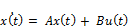(1)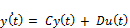(2)

Equation (1) is a system of first-order differential equations and is known as the state equation of the system. The vector x(t) is the state vector, and u(t) is the input vector. Equation (2) is referred to as the output equation. A is called the state matrix, B the input matrix, C the output matrix, and D is the direct transition matrix. One advantage of the state space method is that the form lends itself easily to the digital and analog computation methods of solution. Further, the state space method can be easily extended to the analysis of nonlinear systems. State equations may be obtained from an nth order differential equation or directly from the system model by identifying appropriate state variables. To illustrate the first method, consider an nth order linear plant model described by the differential equation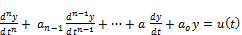(3)

Where y(t) is the plant output and u(t) is the plant input. A state model for this system is not unique but depends on the choice of a set of state variables. A useful set of state variables, referred to as phase variables, is defined as: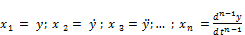(4)

Taking derivatives of the first n- 1 state variables, we have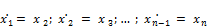(5)

In addition,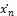comes from rearranging Eq. (3) and substituting from Eq. (4):(6)

In matrix form, this looks like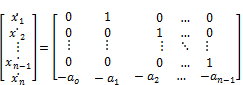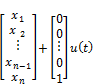(7)

Thus the output equation is simply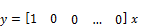(8)

A transfer function that relates an output variable to an input variable represents an nth-order differential equation. In the state-space representation, the nth-order differential equation is written as n first-order differential equations in terms of n state variables . The matrix differential equation= Ax + Bu

used to describe a control system by the state-space method can be solved for the vector of state variables (x) by use of the transfer function matrix. It consists of a matrix of transfer functions that relate the state variables to the inputs. The transfer function matrix serves the same purpose in a multiple-input multiple-output system as the transfer function does for a single-input single-output system. The transfer function matrix is obtained from the matrix differential equatien by application of Laplace transforms. The aim of this work is to study the dynamics of saponification process using state space model. A detail of the reaction and experimental works is shown in .

2. Results and Discussion

The dynamic behavior of saponification process in continuous stirred tank reactor was studied experimentally using step change in the manipulated variables (Ethel acetate flow rate and water coil flow rate (F and Face)) and examine the transient response of sodium acetate and reaction temperature as control variables. The matrics described the dynamics of saponification process as fallow: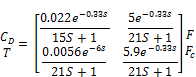The reactions inside the reactor can be described by following reaction :-

Ethyl acetate (A) + Sodium hydroxide (B)à Sodium acetate (D) + Ethanol (E)

A detailed of the system was shown in .

The state space was found by MATLAB and converted to Block diagram to compare with the open loop MIMO system. The above matrics can be represented in MATLAB as fallow:

>> den={ ], ]; ], ]};

>> num={0.022 ,5 ; 0.0056 , 5.9};

>> p=tf (num,den)

p =

From input 1 to output.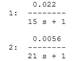From input 2 to output.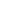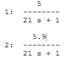Continuous-time transfer function.

>> p.iodelay = [0.33 0.33; 6 0.33];

p =

From input 1 to output.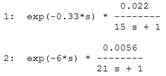From input 2 to output.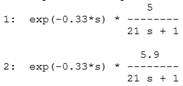Continuous-time transfer function.

>> Sys=ss (p)

sys

a =

 x1 x2 x3 x1 -0.06667 0 0 x2 0 -0.04762 0 x3 0 0 -0.04762

b =

 u1 u2 x1 0.03125 0 x2 0.01563 0 x3 0 1

c =

 x1 x2 x3 y1 0.04693 0 0.2381 y2 0 0.01707 0.281

d =

 u1 u2 y1 0 0 y2 0 0

From Continuous-Time State-Space Model of MATLAB answer is the state space matrics=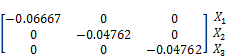+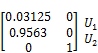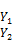=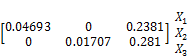Where: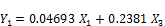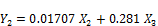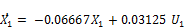(9)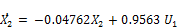(10)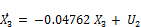(11)

Convert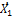to S-Domain from Equation (9)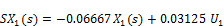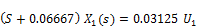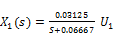(12)

Convert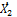to S-Domain from Equation (13)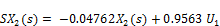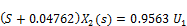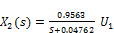(13)

Convert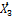to S-Domain from Equation (14)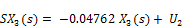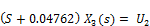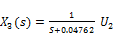(14)

Equations (12), (13) and (14) used to design state space block diagram of CSTR in MIMO system as shown in figure (1).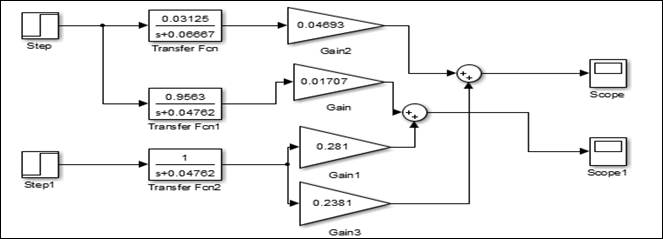Figure 1. Block diagram of state space modeling.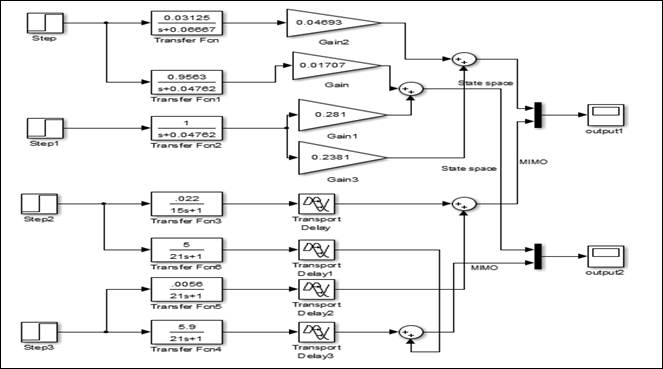Figure 2. Block diagrams of state space model connected with experimental results.Time min

Figure 3. Shows a comparison between the response of the experimental results using 50% step change in feed of ethyl acetate and the state space model.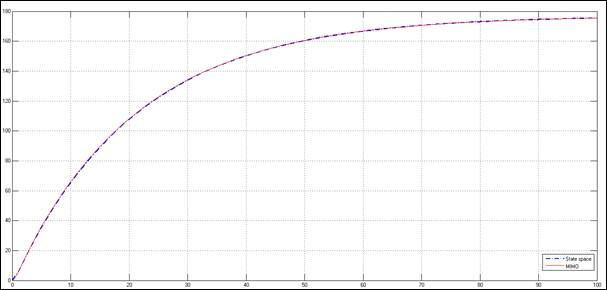Time min

Figure 4. Shows a comparison between the response of the experimental results using 50% step change in water flow rate in coil and the state space model.

Figure 2 shows the block diagram of state space model connected with experimental results to compare the responses. Figure 3 shows a comparison between the response of the experimental results using 50% step change in feed of ethyl acetate and the state space model. Figure 4 shows a comparsion between the response of the experimental results using 50% step change in water flow rate in coil and the state space model.

It's clear that from figures 3 and 4 that great identical was found when using a state space model. Unsatisfied behavior was conducted in previous work in which is a comparison between linearized model and experimental results was shown .

3. Conclusion

A great improvement was found in using a state space model to analysis the dynamic of the saponifecation process compared with the linearized model on which a awkward results was shown.

References

1. http://encyclopedia.che.engin.umich.edu/Pages/Reactors/CSTR/CSTR.html.
2. P.N.Paraskevopoulos, Modern Control Engineering, Control Engineering Series, 2002, P.78.
3. L. Robert Williams and A. Douglas Lawrence, Linear State-Space Control Systems, Ohio University, 2007.
4. L.M. Patnaik, N. Viswanadham, and I.G. Sarma, State Space Formulation of Ammonia Reactor Dynamics, Computers & Chemical Engineering, Volume 4, Issue 4, 1980, P. 215–222.
5. Neil Shephard , Partial Non-Gaussian State Space , Nuffield College, Oxford OX1 1NF, U.K. Biometrika, 1994, P. 115-31.
6. F. Jeremy Burri, D. Stevan Wilson, and Vasilios I. Manousiouthakis, Infinite Dimensional State-Space Approach to Reactor Network Synthesis: Application to Attainable Region Construction, Computers & Chemical Engineering, Volume 26, Issue 6, 2002, P. 849–862.
7. Tapani Raiko and Matti, Learning Nonlinear State-Space Models for Control, Tapani, Neural Networks Research Centre, Helsinki University of Technology, Finland, 2005.
8. C. Or, Jason L. Speyer, and John Kim, State-Space Approximations of the Orr–Sommerfeld System with Boundary Inputs and Outputs, University of California, Los Angeles, California, Journal Of Guidance, Control, And Dynamics, Vol. 33, No. 3, 2010.
9. R.N. Methekar, S.C. Patwardhan, R. Rengaswamy, R.D. Gudi, and V. Prasad, Control of Proton Exchange Membrane Fuel Cells Using Data Driven State Space Models, Chemical Engineering Research and Design, Volume 88, Issue 7, 2010, P. 861–874.
10. J. Siem Koopman, and J.F. Jacques ,Time Series: State Space Methods, International Encyclopedia of the Social & Behavioral Sciences, Second Edition, 2015, P. 354–361.
11. E. Julio Normey-Rico, Control of Dead-time Processes, Advanced Textbooks in Control and Signal processing", 2007, P. 118.
12. R. Donald Coughanowr, Process Systems Analysis and Control, Department of Chemical Engineering, Drexel University, Second Edition, 1991.
13. M. Khalid Mousa, Zaynab Esam Dawood, Study on Dynamics of Continuous Stirred Tank Reactor using Multi Input- Multi Output System,International Journal of Engineering Sciences & Research Technology, 2015.

 Contents 1. 2. 3.
Article ToolsAbstractPDF(1965K)